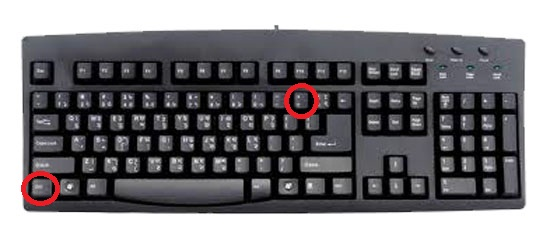# How to get equation in office word 2010?

Introductions:

How to get equation in office word 2010? This is the awesome tips for a computer operator. When he is unable to write some equation on Microsoft word document. You have to need a great idea about equation. Mainly it is need for mathematics students. They are always try to write equation on pain or other programs. But this is the simple tips for all. So, we will learn How to get equation in office word 2010?

For example:

When you are a student of mathematics and need some equation for word file. So, you can get full tips about the equation. See the example below-

1.  a2 , b2 ,
2. H2SO4
3. More equations

## How to get equation in office word 2010?

See the images and get the idea about How to get equation in office word 2010?